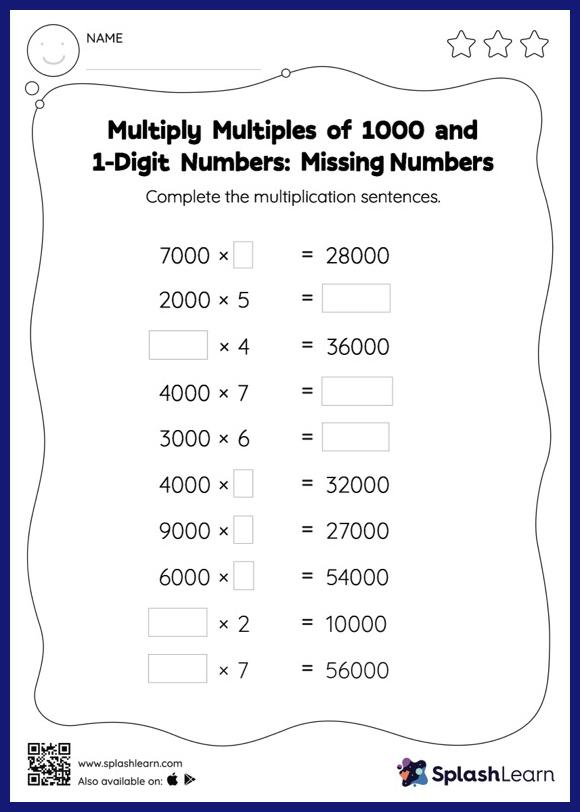# Multiply Multiples of 1000 and 1-Digit Numbers: Missing Numbers Worksheet

Home > Multiply Multiples of 1000 and 1-Digit Numbers: Missing NumbersLearners hone their math skills with this multiply multiples of 1000 and 1-digit numbers worksheet. As the worksheet uses a horizontal format, it allows for more creativity when it comes to how the student solves the problem. In contrast, in the vertical format, students usually employ the standard method to solve.